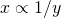# Ratio and Proportion

Ratio – the ratio of two quantity a & b in the same unit is the fractionand we write it a:b
In a ratio a:b we call a as the first term or antecedent and b the second term or consequent.

Rule– the multiplication or division of each term of a ratio by the same non zero number does not effect the ratio.
Example
4:5 = 8:10= 12:15, also 12:15 = 8:10

Proportion– the quantity of two ratios is called proportion
a:b = c:d we write a:b ::c:d and we say that a,b,c, d, are in proportion. Here a and d are called extremes while b & c are called mean term

Product of means = product of extreme
Thus a:b::c:d
(b×c) = (a×d)

Third proportion
If a:b=b:c, then c is called the third proportion to a & b.
4:8::8:16 – 16 is called third proportion.

Continued proportion e.g. 4:3::8:16
Forth proportion
If a:b = c:d then d is called the fourth proportion to a,b,c

Mean proportion
Mean proportion between a & b is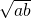4:2::8:16 or 82 = 4×16
8 is mean proportion.

Comparison of ratio

a:b>c:d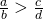Compound ratio– duplicate ratio a:b is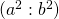Sub duplicate ratio– a:b is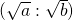Triplicate ratio – a:b isSub triplicate ratio– a:b is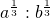If,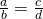thenVerification:-
1) We say that x is directly proportional to y, if x= ky for some constant K
then we write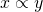2) We say that x is inversely proportional to y, if xy = k for some constant K
then we write